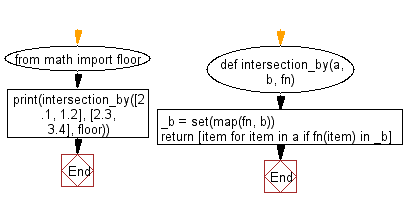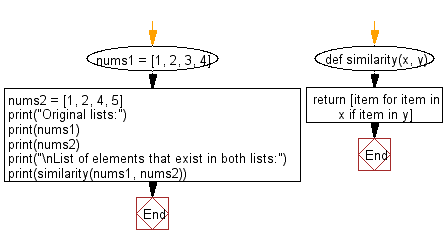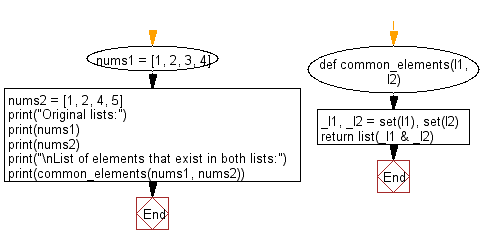﻿ Python: Get a list of elements that exist in both lists - w3resource# Python: Get a list of elements that exist in both lists

## Python List: Exercise - 226 with Solution

Write a Python program to get a list of elements that exist in both lists, after applying the provided function to each list element of both.

• Create a set, using map() to apply fn to each element in b.
• Use a list comprehension in combination with fn on a to only keep values contained in both lists.

Sample Solution-1:

Applying a given function:

Python Code:

``````def intersection_by(a, b, fn):
_b = set(map(fn, b))
return [item for item in a if fn(item) in _b]
from math import floor
print(intersection_by([2.1, 1.2], [2.3, 3.4], floor))
```
```

Sample Output:

```[2.1]
```

Flowchart:## Visualize Python code execution:

The following tool visualize what the computer is doing step-by-step as it executes the said program:

Sample Solution-2:

Without applying any function:
Use a list comprehension on x to only keep values contained in both lists.

Python Code:

``````def similarity(x, y):
return [item for item in x if item in y]
nums1 = [1, 2, 3, 4]
nums2 = [1, 2, 4, 5]
print("Original lists:")
print(nums1)
print(nums2)
print("\nList of elements that exist in both lists:")
print(similarity(nums1, nums2))
```
```

Sample Output:

```Original lists:
[1, 2, 3, 4]
[1, 2, 4, 5]

List of elements that exist in both lists:
[1, 2, 4]
```

Flowchart:## Visualize Python code execution:

The following tool visualize what the computer is doing step-by-step as it executes the said program:

Sample Solution-3:

Without applying any function:

• Create a set from a and b.
• Use the built-in set operator & to only keep values contained in both sets, then transform the set back into a list.

Python Code:

``````def common_elements(l1, l2):
_l1, _l2 = set(l1), set(l2)
return list(_l1 & _l2)
nums1 = [1, 2, 3, 4]
nums2 = [1, 2, 4, 5]
print("Original lists:")
print(nums1)
print(nums2)
print("\nList of elements that exist in both lists:")
print(common_elements(nums1, nums2))
```
```

Sample Output:

```Original lists:
[1, 2, 3, 4]
[1, 2, 4, 5]

List of elements that exist in both lists:
[1, 2, 4]
```

Flowchart:## Visualize Python code execution:

The following tool visualize what the computer is doing step-by-step as it executes the said program:

Python Code Editor:

Have another way to solve this solution? Contribute your code (and comments) through Disqus.

What is the difficulty level of this exercise?

Test your Python skills with w3resource's quiz

﻿

## Python: Tips of the Day

Floor Division:

When we speak of division we normally mean (/) float division operator, this will give a precise result in float format with decimals.

For a rounded integer result there is (//) floor division operator in Python. Floor division will only give integer results that are round numbers.

```print(1000 // 300)
print(1000 / 300)```

Output:

```3
3.3333333333333335```